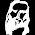## Saturday, June 8, 2013

### Problem 886: Right Triangle, Incenter, Angle Bisector, Perpendicular, 45 Degrees, Concyclic Points, Isosceles Right Triangle

Geometry Problem. GeoGebra, HTML5 Animation for iPad and Nexus.

Level: Mathematics Education, High School, Honors Geometry, College.

Click the figure below to see the complete problem 886.1.∵ ΔAEB≡ΔAEG and ΔCDB≡ΔCDF
∴ AB=AG and CB=CF
i.e. ΔABG and ΔCBF are isosceles.

That means the figure is essentially equivalent to that in Problem 792.

That is, if we draw BM⊥AC at M,
then H and L are the incenters of ΔABM and ΔCBM, respectively.

⇒ BF bisects ∠ABM and BG bisects ∠CBM
⇒ ∠FBG=45°

∵ ΔABG and ΔCBF are isosceles
∴ AJ⊥BG and CK⊥BF
∴ BJIK concyclic with diameter BI.

Also, it follows from Problem 792,
that FHILG concyclic, with diameter FG.

∵ FG is the diameter of circle FHILG
∴ FI⊥IG

From Problem 790, IB=IF=IG.
Thus, ΔFIG is isosceles right triangle.

2.Draw altitude BP
1. We have FP/FA=DB/DA=BC/AC = cos(C)=cos(∠ABP)=BP/AB ( property of angle bisector)
So BF is angle bisector of ∠ (ABP) .
Similarly BG is angle bisector of ∠ (CBP) .
So ∠ (FBG)=1/2*(∠ABC)= 45 degrees
2. ∠ (IAC)+ ∠ (ICA)= ½(∠BAC+ ∠ BCA)= 45 so ∠ (AIC)=135 => B, K, I, J is concyclic
3. Note that DC is a perpendicular of BF => BF ⊥DC and LB=LF
Triangles BKl, FKL are isosceles => ∠BLK=∠KLF= 45
So ∠FLG= 90 .
Similarly FH is perpendicular to BH.
4. Since I is the intersection of perpendicular bisector of BF and BG so I is circumcenter of triangle FBG
So IF= IG and % FIG= 90 and F, H, I, L, G is concyclic and FIG is right isosceles triangle